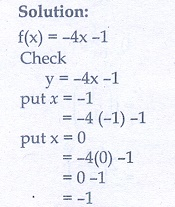Home | | Maths 10th Std | Exercise 1.5: Composition of Functions

# Exercise 1.5: Composition of Functions

Maths Book back answers and solution for Exercise questions - Mathematics : Function: Composition of Functions: Exercise Problem Questions with Answer

Exercise 1.5

1. Using  the functions f  and  g  given  below, find o and o . Check whether f o o f.

(i) (x) = − 6, g(x) = x2

(ii) (x) = 2/x, g(x) = 2x2 – 1

(iii) (x) =g(x) = 3 – x

(iv) (x) = 3 + x, g(x) = – 4

(v) (x) = 4x2 − 1, g(x) = 1 + x2. Find the value of k, such that o o f

(i) f (x) = 3+ 2, g(x) = 6– k

(ii) (x) = 2− kg(x) = 4+ 53. If (x) = 2− 1, g(x) = [x+1]/2 show that o o x4. (i) If (x) = x2 − 1, g(x) = − 2 find a, if o (a) = 1.

(ii) Find k, if (k) = 2− 1 and o (k) = 5 .5. Let AB, N and a function → be defined by (x) = 2+ 1 and → C be defined by g(x) = x2 . Find the range of o and o .6. Let (x) = x2 − 1 . Find (i) o (ii) o o f7. If R → R and R → R are defined by (x) = x5 and g(x= x4 then check if f, are one-one and o is one-one?8. Consider the functions (x), g(x), h(x) as given below. Show that

(o go o (o h) in each case.

(i) f (x) = − 1, g(x) = 3+ 1 and h(x) = x2

(ii) (x) = x2, g(x) = 2x and h(x) = + 4

(iii) (x) = − 4, g(x) = x2 and h(x) = 3– 59. Let = {(−1, 3),(0,−1),(2,−9)} be a linear function from Z into Z. Find (x).10. In electrical circuit theory, a circuit C(t) is called a linear circuit if it satisfies the superposition  principle  given  by  (at1 + bt2 ) = aC (t1) + bC(t2),  where  a,are constants. Show that the circuit (t) = 3is linear.